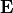# YoungModulus¶

Type: 2-Tensor, or section [v_1, …, v_9] -/- optional

Defines Young’s modulusalso known as tensile modulus or elastic modulus. Units are pascals,Youngs’ modulus connects tensile stress of a body,(normal force per area), to tensile strain of the body,(relative length change):.

A constant Young’s modulus can be defined by assigning a rank-2 tensor:

Material {
# defines a constant Young's modulus
YoungModulus =
[..., ..., ...
..., ..., ...
..., ..., ...]
}


Assign a scalar for an isotropic Young’s modulus.

For more general cases, the Young’s modulus may be given as a section in order to deal with space, time, and parameter dependent definitions:

# define the Young's modulus as a section
YoungModulus {
Python {...}
...
}


Field definitions within the section YoungModulus are summed up. Consult the subsequent sections to see which types of field definitions are allowed.

Note

Setting the Young’s modulus together with the Poisson ratio , see PoissonRatio, will implicitly define a stiffness matrix. For details, we refer to Stiffness.